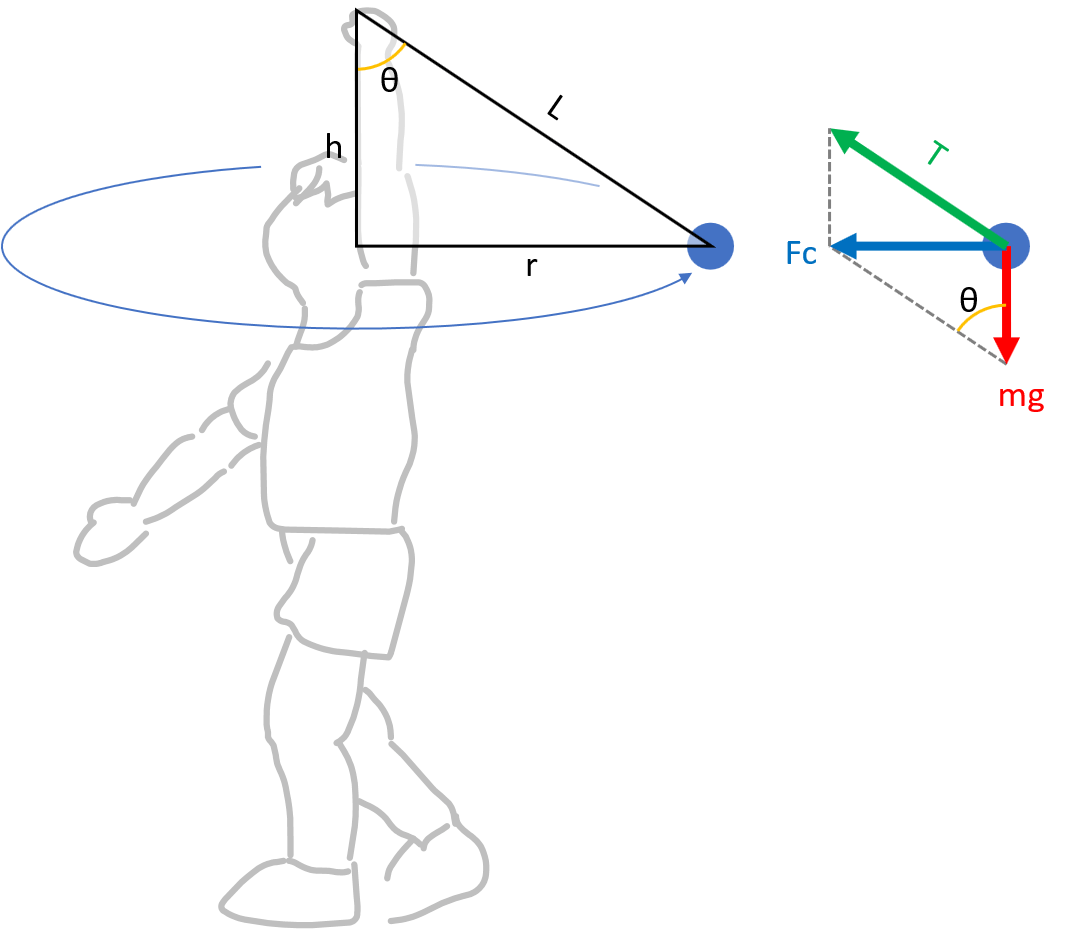# Conical pendulum

## 3 forces acting on a conical pendulum

• Gravity(=mg): The force the Earth pulls.
• Tension: The force applied to both ends of the thread. Tension is always directed to the center from both ends.
• Centripetal force(Fc): The force that maintains circular motion. The centripetal force always toward the center of rotation.$F_{c} = mr \omega ^{2} = mr {( \frac{2\pi }{T})}^2$

ω: Angular velocity of rotational motion (rad/s)
T: period of rotational motion (seconds)

## Find the period of a conical pendulum

Consider a conical pendulum with a line length ‘L’ and a rotation radius ‘r.’
Centripetal force(Fc) is the result of gravity and tension. Also, the centripetal force is perpendicular to gravity. From this, the following equation is calculated.

$F_{c} = mg \cdot tan \theta$

Substituting the definition of centripetal force comes the following equation.

$mr {( \frac{ 2 \pi }{T})}^2 = mg \cdot tan \theta$

${( \frac{ 2 \pi }{T})}^2 = \frac {g }{r} \cdot tan \theta$

Since it is $$tan \theta = \frac{r}{h}$$, we can make it a bit simpler.

${( \frac{ 2 \pi }{T})}^2 = \frac {g}{h}$

Solving the above equation for the period T, It becomes as follows.

$T = 2 \pi \sqrt{\frac {h}{g}}$